# Exam-Style Questions.

## Problems adapted from questions set for previous Mathematics exams.

### 1.

GCSE Higher

This Cumulative Frequency graph shows the heights of 58 Scouts. Work out an estimate for the number of these Scouts with a height greater than 160cm.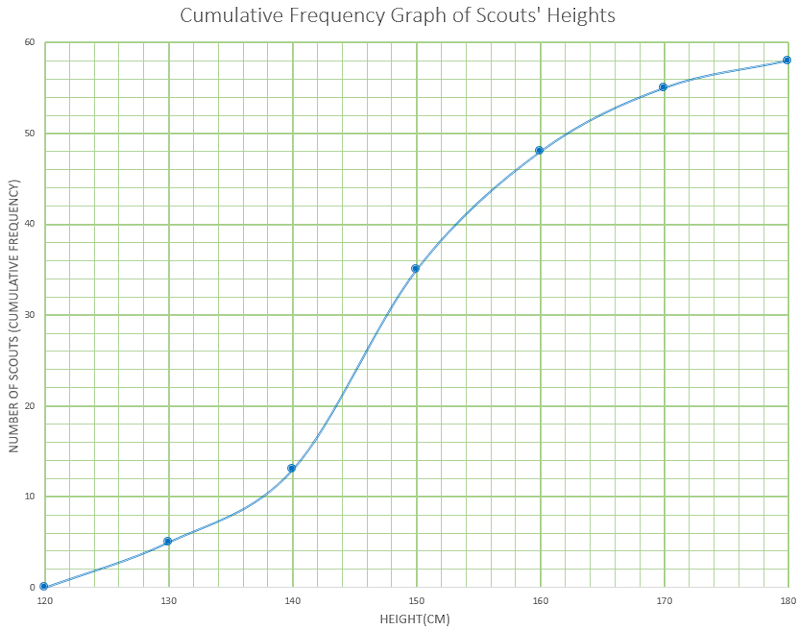### 2.

GCSE Higher

The grouped frequency table gives information about the times, in minutes, that 90 commuters take to get home from work.

Time (t minutes)Frequency
$$0 \lt t \le 10$$6
$$10 \lt t \le 20$$32
$$20 \lt t \le 30$$22
$$30 \lt t \le 40$$10
$$40 \lt t \le 50$$10
$$50 \lt t \le 60$$7
$$60 \lt t \le 70$$3

(a) Complete the cumulative frequency table.

Time (t minutes)Cumulative frequency
$$0 \lt t \le 10$$
$$0 \lt t \le 20$$
$$0 \lt t \le 30$$
$$0 \lt t \le 40$$
$$0 \lt t \le 50$$
$$0 \lt t \le 60$$
$$0 \lt t \le 70$$

(b) On the grid, draw the cumulative frequency graph for this information.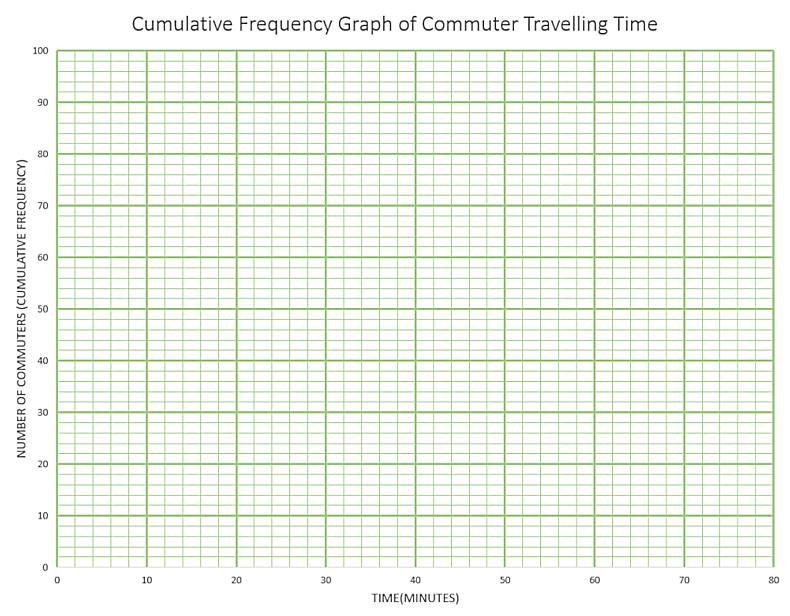(c) Use your graph to find an estimate for the percentage of these commuters who take more than 45 minutes to get home from work.

### 3.

GCSE Higher

The table shows information about the heights of 85 stalagmites.

Height ($$h$$ cm) Frequency
$$10\lt h \le 15$$9
$$15\lt h \le 20$$13
$$20\lt h \le 25$$18
$$25\lt h \le 30$$22
$$30\lt h \le 35$$15
$$35\lt h \le 40$$8

(a) Find the class interval that contains the median.

(b) On the grid below, draw a frequency polygon for the information in the table.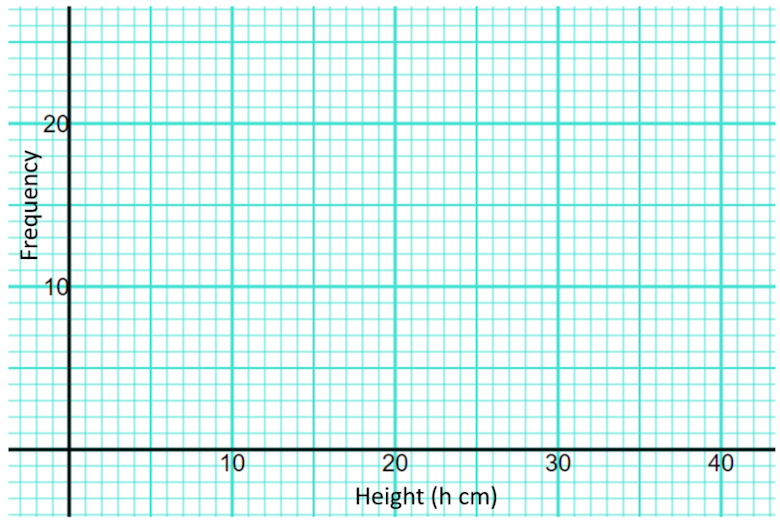### 4.

GCSE Higher

The table shows the marks earned by 200 students taking a Maths exam.

 Mark (n) $$0\lt n \le 10$$ $$10\lt n \le 20$$ $$20\lt n \le 30$$ $$30\lt n \le 40$$ $$40\lt n \le 50$$ $$50\lt n \le 60$$ $$60\lt n \le 70$$ $$70\lt n \le 80$$ Frequency 3 7 33 42 54 35 20 6

(a) Use the data in the table above to complete the following cumulative frequency table

 Mark (n) $$n \le 10$$ $$n \le 20$$ $$n \le 30$$ $$n \le 40$$ $$n \le 50$$ $$n \le 60$$ $$n \le 70$$ $$n \le 80$$ Cumulative Frequency 200

(b) Draw the cumulative frequency curve on graph paper.

(c) Use your graph to estimate the lowest mark that B grade will be awarded for.

### 5.

GCSE Higher

The table shows the amount of time, in months, taken to sell houses on the Happyland housing estate.

 Time (m months) Frequency 0 < m ≤ 2 10 2 < m ≤ 5 21 5 < m ≤ 10 25 10 < m ≤ 15 20 15 < m ≤ 20 25

(a) Draw a histogram for the information in the table.

(b) Find an estimate for the median.

### 6.

GCSE Higher

This histogram shows information about the ages of 317 people attending a charity concert. The cost of tickets for children under 15 years of age are half price. If a full price ticket was £18, estimate the total income of all 317 ticket sales.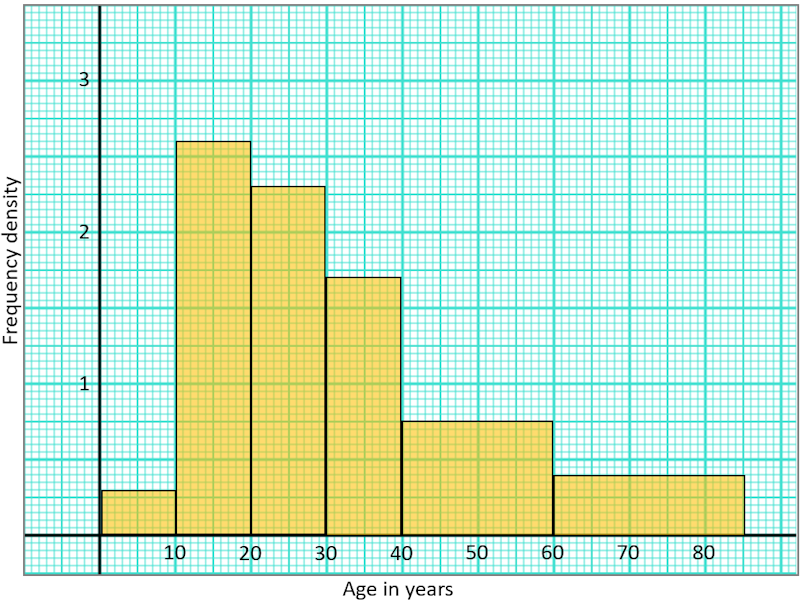### 7.

IB Analysis and Approaches

A TV company surveyed 88 of its employees to find out how much time they spend travelling to work on a given day. The results of the survey are shown in the following cumulative frequency diagram.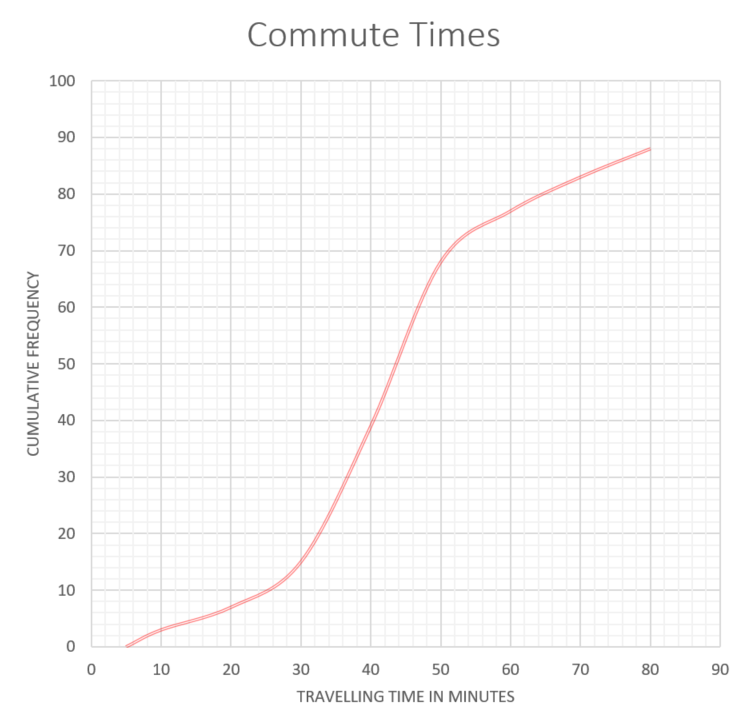(a) Find the median number of minutes spent travelling to work.

(b) Find the interquartile range.

(c) Find the number of employees whose travelling time is within 20 minutes of the median.

(d) Only 10% of the employees spent less than k minutes travelling to work. Find the value of k.

The results of the survey can also be displayed on the following box-and-whisker diagram.(e) Write down the value of a.

(f) Find the value of b.

(g) Travelling times of less than p minutes are considered outliers. Find the value of p .

### 8.

IB Studies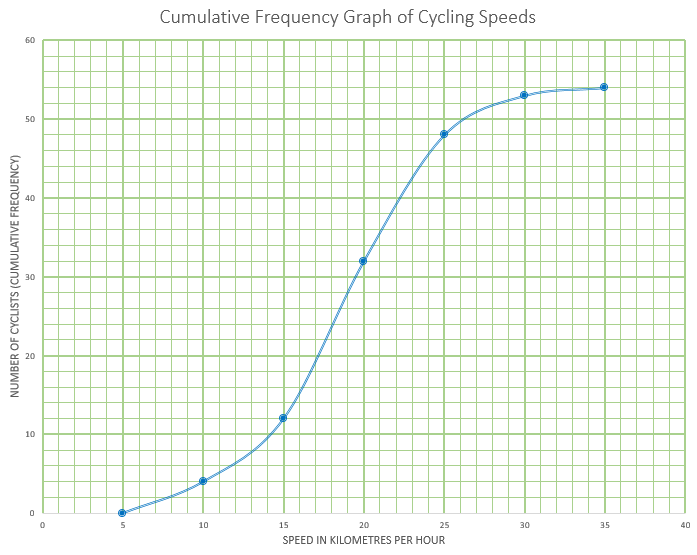This cumulative frequency graph shows the speeds in kmh-1 of cyclists passing a certain point on a race track.

(a) Estimate the minimum possible speed of one of these cyclists.

(b) Find the median speed of the cyclists.

(c) Write down the 65th percentile.

(d) Calculate the interquartile range.

(e) Find the number of these cyclists that were travelling faster than 22 kmh-1

The table shows the speeds of these cyclists.

Speed of Cyclists (s)Number of Cyclists
$$0 \lt s \le 5$$0
$$5 \lt s \le 10$$$$a$$
$$10 \lt s \le 15$$8
$$15 \lt s \le 20$$20
$$20 \lt s \le 25$$16
$$25 \lt s \le 30$$5
$$30 \lt s \le 35$$$$b$$

(f) Find the value of $$a$$ and of $$b$$

(g) Write down the modal class.

(h) Write down the mid-interval value for the modal class.

(i) Use your graphic display calculator to calculate an estimate of the mean speed of these cyclists.

(j) Use your graphic display calculator to calculate an estimate of the standard deviation of the speeds of these cyclists.

If you would like space on the right of the question to write out the solution try this Thinning Feature. It will collapse the text into the left half of your screen but large diagrams will remain unchanged.

The exam-style questions appearing on this site are based on those set in previous examinations (or sample assessment papers for future examinations) by the major examination boards. The wording, diagrams and figures used in these questions have been changed from the originals so that students can have fresh, relevant problem solving practice even if they have previously worked through the related exam paper.

The solutions to the questions on this website are only available to those who have a Transum Subscription.

Exam-Style Questions Main Page

Search for exam-style questions containing a particular word or phrase:

To search the entire Transum website use the search box in the grey area below.